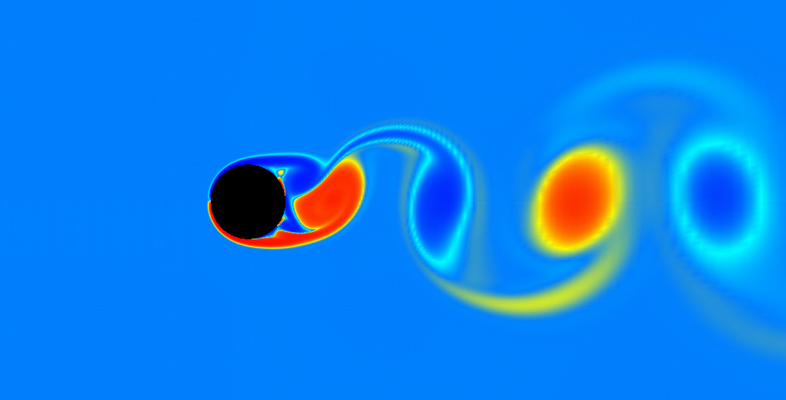Science, Maths & Technology

### Become an OU studentKinematics of fluids

Start this free course now. Just create an account and sign in. Enrol on the course to track your learning.

# Kinematics of fluids

## Introduction

Please note: a Statement of Participation is not issued on this course.

This free OpenLearn course, Kinematics of fluids, is an extract from the Open University course MST326 Mathematical methods and fluid mechanics [Tip: hold Ctrl and click a link to open it in a new tab. (Hide tip)] , a third level applied mathematics course which provides an introduction to the subject of fluid mechanics. Half of this course is about modelling simple fluid flows; the other half is about mathematical methods. The fluid mechanical aspects of the course will give you a good understanding of modelling in the context of fluids.

Kinematics of fluids consists of material from MST326 Unit 5, Kinematics of fluids, and has five sections in total. You should set aside about three to four hours to study each of the sections; the whole extract should take about 16 hours to study. The extract is a small part (around 8%) of a large course that is studied over eight months, and so can give only an approximate indication of the level and content of the full course.

The aim of this extract is to introduce the equations of streamlines and pathlines, develop the concept of a stream function as a method of describing fluid flows and formulate Euler’s equation of motion for an inviscid fluid. The extract is relatively self-contained and should be reasonably easy to understand for someone with a sound knowledge of relevant mathematics, such as could be gained from Open University level 2 study of applied mathematics including vector calculus and differential equations.

Mathematical/statistical content at the Open University is usually provided to students in printed books, with PDFs of the same online. This format ensures that mathematical notation is presented accurately and clearly. The PDF of this extract thus shows the content exactly as it would be seen by an Open University student. However, the extract isn't entirely representative of the module materials, because there are no explicit references to use of the MST326 software or to video material (although please note that the PDF may contain references to other parts of MST326). In this extract, some illustrations have also been removed due to copyright restrictions.

Regrettably, mathematical and statistical content in PDF form is not accessible using a screenreader, and you may need additional help to read these documents.

Sections 1–3 deal with the basic kinematics of two-dimensional fluid flows.

Section 1 introduces the differential equations for pathlines and streamlines.

Section 2 introduces a scalar ﬁeld, called the stream function, which for an incompressible ﬂuid provides an alternative method of modelling the ﬂow and ﬁnding the streamlines.

Sections 2 and 3 derive the stream functions for several simple two-dimensional ﬂow types (the uniform ﬂow, source, doublet and vortex), and suitable combinations of these are used to model more complicated ﬂows.

Section 4 introduces the idea of differentiation following the motion, which is necessary for the development of Euler’s equation in Section 5.